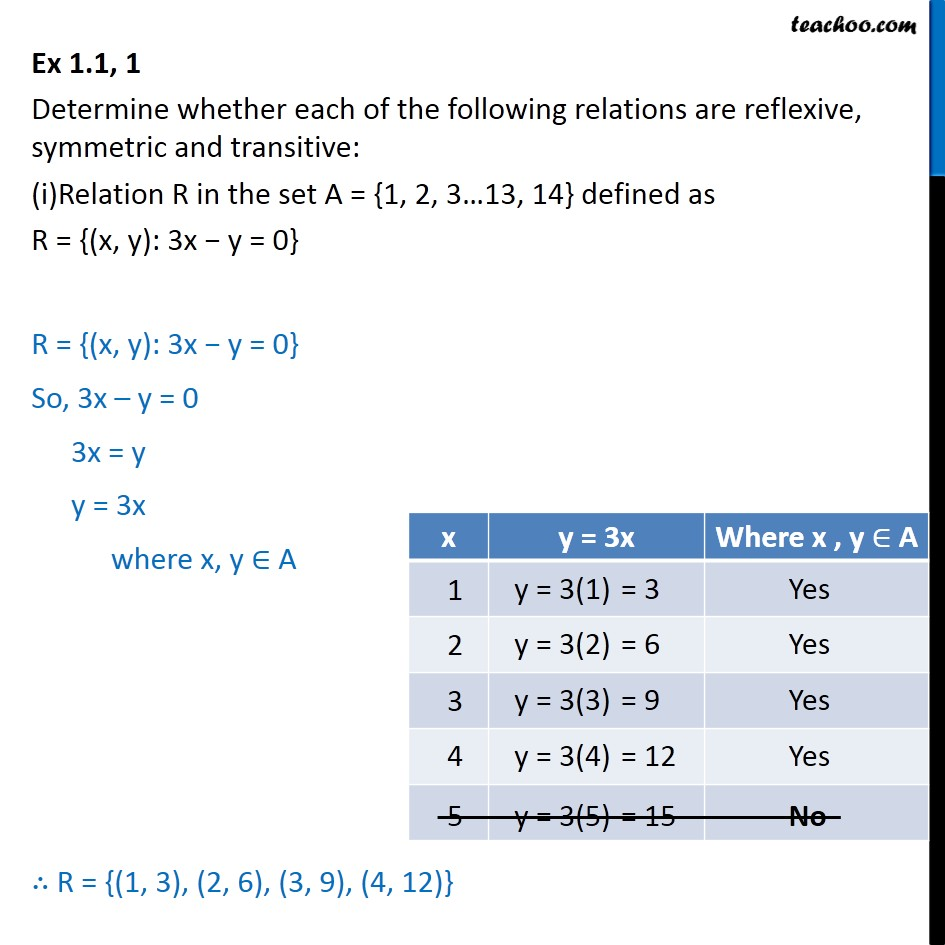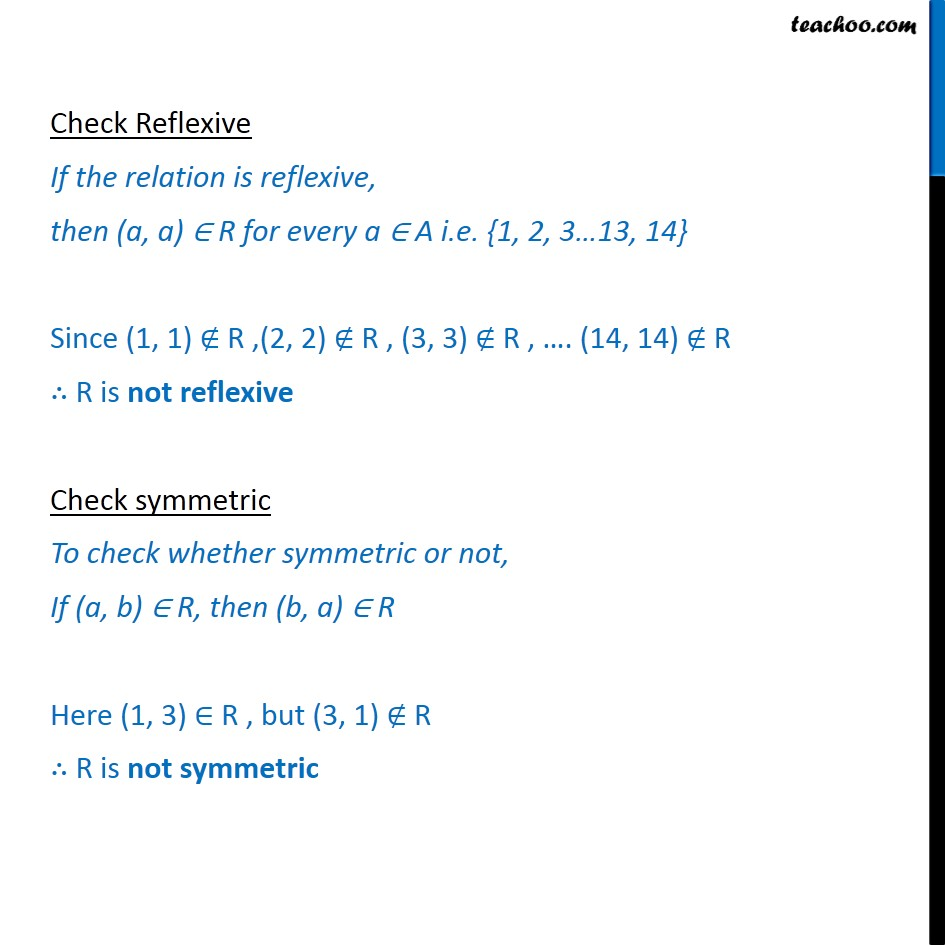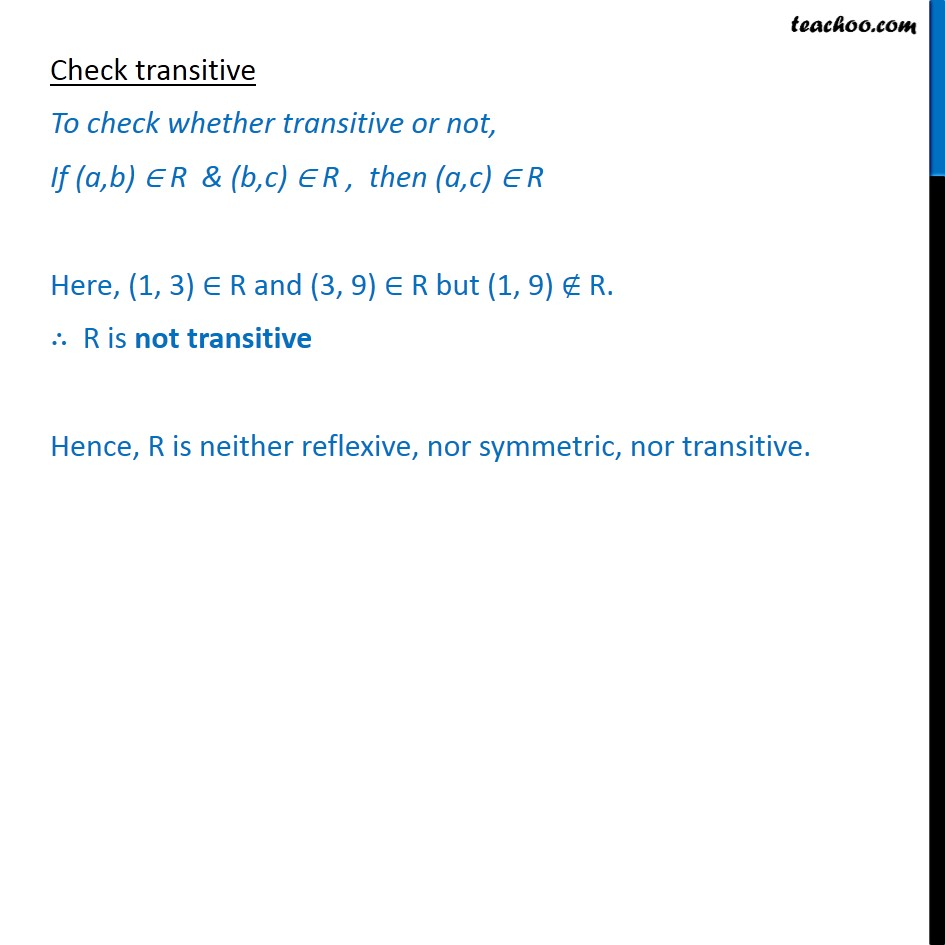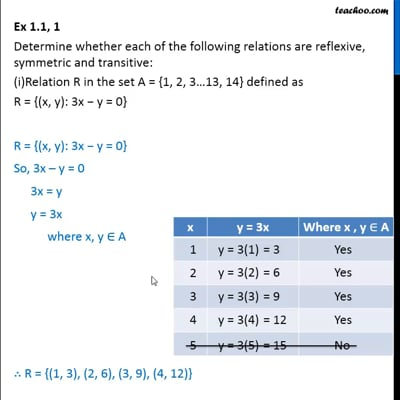To prove relation reflexive, transitive, symmetric and equivalent

Chapter 1 Class 12 Relation and Functions
Concept wiseThis video is only available for Teachoo black users

Introducing your new favourite teacher - Teachoo Black, at only ₹83 per month

### Transcript

Ex 1.1, 1 Determine whether each of the following relations are reflexive, symmetric and transitive: (i) Relation R in the set A = {1, 2, 3 13, 14} defined as R = {(x, y): 3x y = 0} R = {(x, y): 3x y = 0} So, 3x y = 0 3x = y y = 3x where x, y A R = {(1, 3), (2, 6), (3, 9), (4, 12)} Check Reflexive If the relation is reflexive, then (a, a) R for every a A i.e. {1, 2, 3 13, 14} Since (1, 1) R ,(2, 2) R , (3, 3) R , . (14, 14) R R is not reflexive Check symmetric To check whether symmetric or not, If (a, b) R, then (b, a) R Here (1, 3) R , but (3, 1) R R is not symmetric Check transitive To check whether transitive or not, If (a,b) R & (b,c) R , then (a,c) R Here, (1, 3) R and (3, 9) R but (1, 9) R. R is not transitive Hence, R is neither reflexive, nor symmetric, nor transitive.